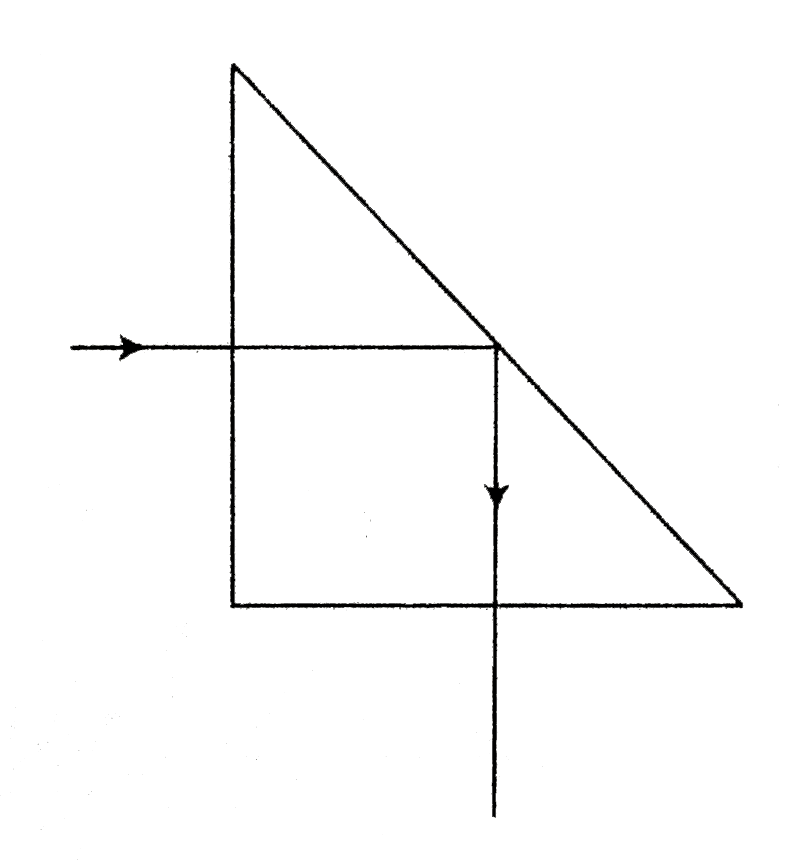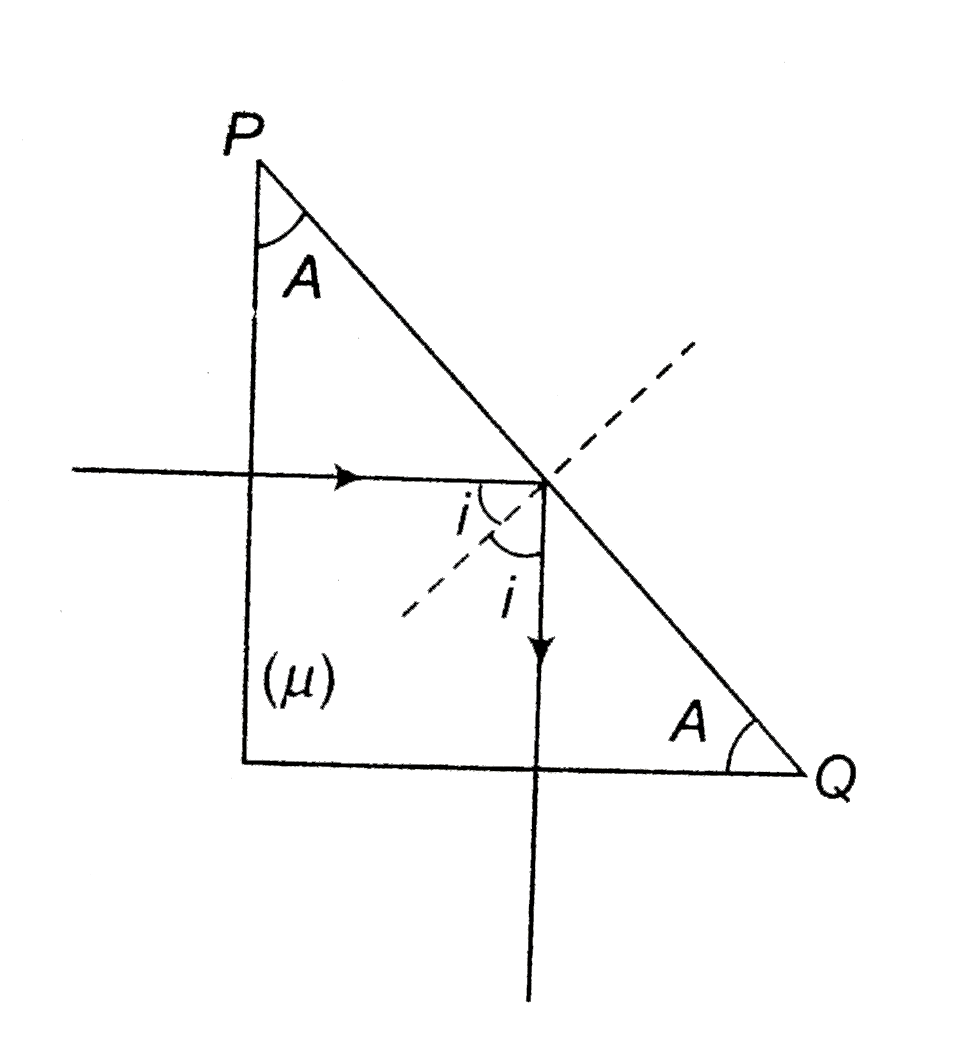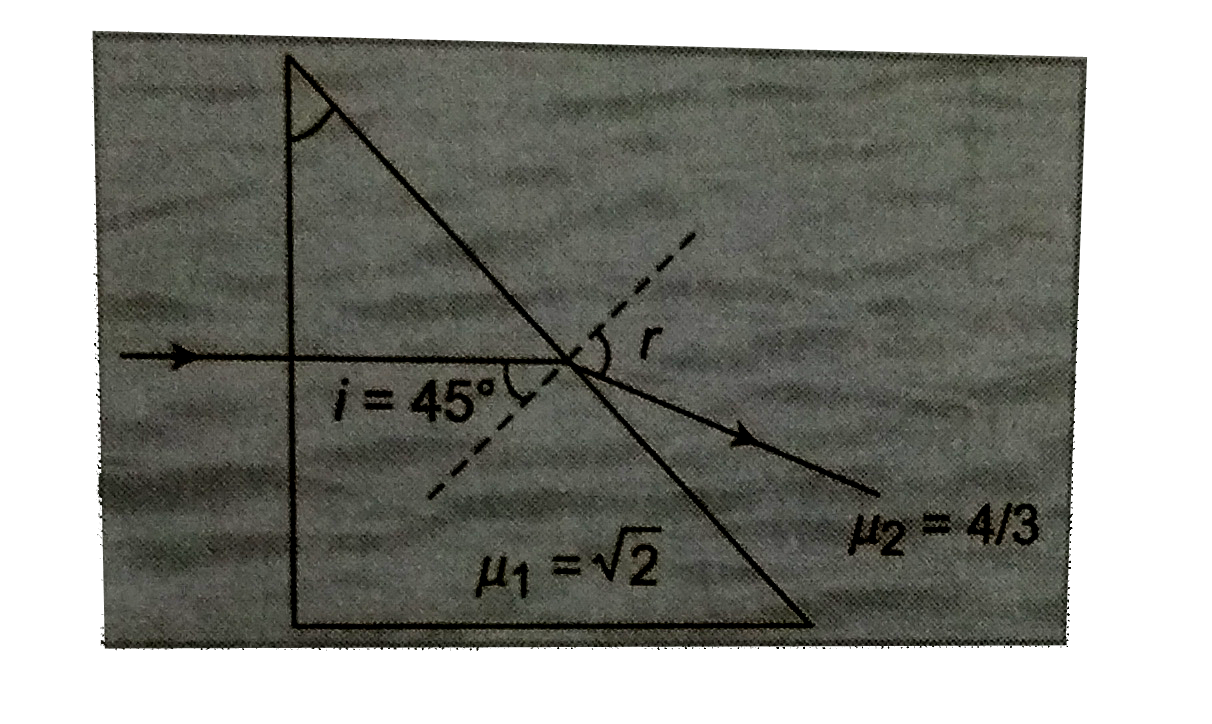# A ray of light incident normally on one of the faces of a right-angle isosceles prism is found to be totally reflected as shown in the figure. What is

54 views
in Physics
closed
A ray of light incident normally on one of the faces of a right-angle isosceles prism is found to be totally reflected as shown in the figure. What is the minimum value of the refractive index of the material of the prism? When the prism is immersed in water, trace the path of the emergent ray for the same incident ray, indicating the values of all the angles (mu_omega=4//3).by (84.0k points)
selectedsinC=1//mu, A=45^@
For T.I.R. on PQ
igeC
AgeC
sinAgesinC
sin45^@ge1//muimplies1/sqrt2ge1/mu
mugesqrt2
mu_(min)=sqrt2mu_1sin45^@=mu_2sinr
sqrt2xx1/sqrt2=4/3sinr
sinr=3/4
r=sin^-1(3/4)